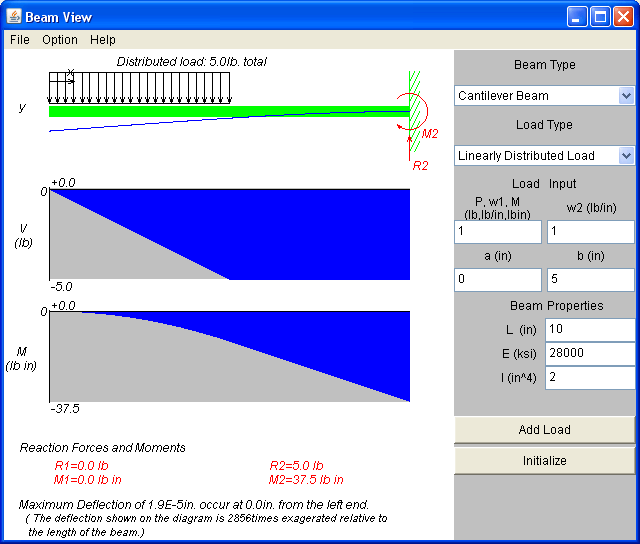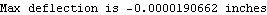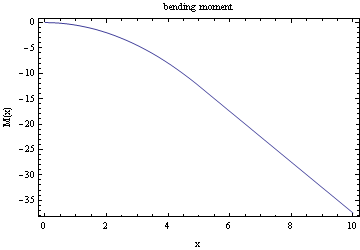Solving beam deflection problems using the moment-deflection approach and using the Euler-Bernoulli approach

by Nasser M. Abbasi
November 2009

Introduction

These are problems in beam deflection showing how to use Mathematica to solve them.

Problem 1

This is problem 9-3, page 551, from bok Problem Solvers, strength of materials and mechanics of materials by REA. I show here how to solve this problem using Mathematica.

Start by setting up the moment deflection equation for the Euler beam E I  y''[x]=M(x) , this equation is found for both halves of the beam resulting in 2 solutions,and.

On the right side, boundary condition is thatand on the left side, then we need an additional 2 boundary conditions, for this, use the  continuity  conditions at L/2 where we setandand now we have the complete solutions.

For the left half of beam, it is easily found thatand for the right halfFindUse first boundary conditionFindUse boundary conditionWe see from the above, that we have 2 additional constants of integrations to solve for. c2 and c4. To solve for these, we use the continuity conditions at L/2All the integration of constants now found, we can now plot moment diagrams, shear diagram, and deflection diagramsAssign some values for E, I, L and w and do the plots. PLot the deflectionPlot the bending momentPlot the shear diagramSolving the same problem directly from the Euler-Bernoulliorder ODE

The above approach (using the Moment-deflection ODE) is a standard approach to solve deflection beam problems. However, we can also use the 4th order Euler beam equation direclty as follows. One needs to make sure that the load on the RHS of this ODE is the load per unit length only, i.e. w in this problem. Notice the use of the unit step function since w only acts on half the beam.Assign some values for E, I, L and w and do the plots. PLot the deflectionWe see that we obtain the same result as earlier.

Plot the bending momentPlot the shear diagramProblem 2

In this problem. I set up a cantilever beam and first solved it using the Java beam applet that can be accessed  here
and then I solved this problem using Mathematica below, showing that I get the same answer as shownThe boundary conditions to use are: y[L] == 0, y'' == 0, y''' == 0, y'[L] == 0Out=Assign same values as shown above for E, I, L and w and do the plots.

In:=Out=Plot the bending moment

In:=Out=Plot the shear diagram

In:=Out=